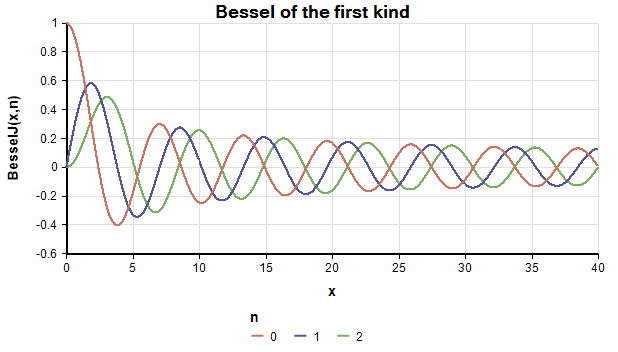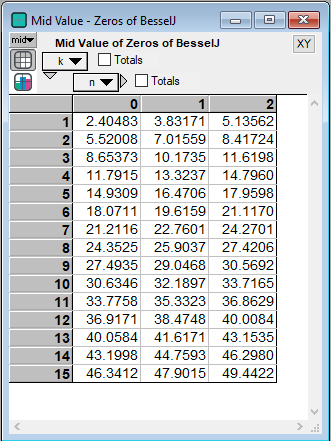# Bessel Functions

(Redirected from BesselI)

Bessel Functions are used in engineering models to describe harmonic vibrations in cylindrical systems, such as electromagnetic waves in a cylindrical waveguide, sound vibrations in a circular membrane, or heat conduction in a cylindrical object. Analytica exposes the following Bessel functions:

BesselJ(x, n): Bessel function of the first kind
BesselY(x, n): Bessel function of the second kind
BesselK(x, n): Modified Bessel function of the first kind
BesselY(x, n): Modified Bessel function of the second kind

In each case, «n» is the order of the Bessel function and can be zero or greater. Analytica will compute these Bessel functions with non-integer «n» when «x» is non-negative. A Bessel function applied to a negative value of «x» when «n» is non-integer would result in a complex number -- in Analytica, a warning results and NaN is returned.

(New to Analytica 6.0) The zeros (roots) of a Bessel function can be obtained from:

BesselJZero(n,k)
BesselYzero(n,k)

where «n» is the order and «k» is the zero number.

## Library

BesselJ(x,n)BesselJZero(n,k)